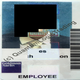Panjang

APPLE LONG: \$151-\$182 - CYCLICAL ANALYSIS & REGRESSION FORECAST

NASDAQ:AAPL   Apple Inc.
Dilihat 312 kali
312
Analysing Apple's ( AAPL ) historical cyclical price movements and using the +/- 2SD of the linear regression to forecast a naive regression price for the next extension phase.

* Extension leg Regression Forecast*

1. For leg A (Extension Leg 1) we use a start point of \$12.5 or \$33 (phase doesnt have a clear start), or we could assume a mean value of (12.5+33)/2= \$22.75.

- Leg A is then, \$12.5, \$23.5 or \$33 divided by \$100, which means Leg A is a price increase of = 700%, 310% or 200%

2. For Leg C (Extension Leg 2) the price increased from \$55 to \$134.5 which is a 145% increase.

3. For Foretasted Leg E (Extension Leg 3), we start at \$89 and we derive the price "%" increase by:

- Using the regression of the price increase % from Leg A to Leg C e.g. 145%/700%= 21%; 145/310 = 46%; 145/200= 73%, so this means for each of the calculations we can then assume each is the regression growth differential from Leg C's 145% increase to foretasted Leg E's "%" increase

4. e.g. Foretasted Leg E/ Extension Leg 3:
21% of 145% = 31% increase; \$89 * 31% = \$117
or 46% of 145% = 67% increase ; \$89* 67% * \$89= \$151
or 73% of 145% = 106% increase; \$89 * 106% = \$182

- Thus Apples Leg E/ Extension Leg 3's Naive Regression Forecast = between \$151 and \$182
* As shown on graph.

Furthermore, another interesting statistical measure for apples 10year/ 120 Month +/-2SD channel was that the Pearsons R was 0.95. This means that the linear correlation between Apples Price over the measured time period was 95%. 95% of all values observed lie averagely on its linear regression line (middle line of the Stan Dev channel) - en.wikipedia.org/wiki/Pearson_product-moment_correlation_coefficient

- Having such a high Peasons R means the regression line holds true for 95% of past data and therefore is MAY also include 95% of future data thus extrapolating the linear line (or using basic regressions as i have done) is of some statistical significance.
A Pearsons R coefficient of 0.3 means there is little positive correlation between Price and Time, thus extrapolating prices through time using basic regressions/ forecasts is much less statistically prudent, since only 30% of past data correlated about the linear regression line.